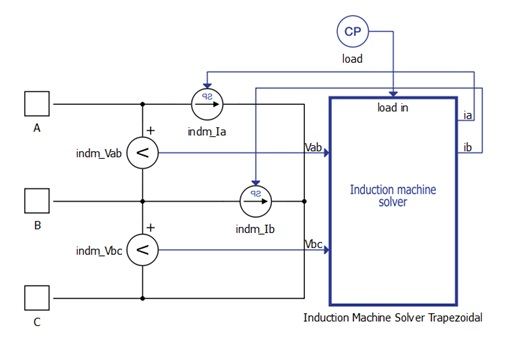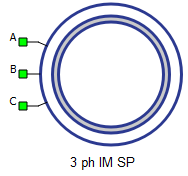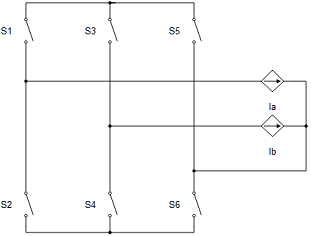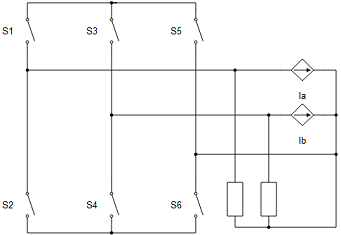# Three Phase Squirrel Cage Induction Machine (SP)

Description of the Three Phase Squirrel Cage Induction Machine (SP) component in Schematic Editor.

This machine is an alternative implementation of the Three Phase Squirrel Cage Induction Machine which relies on Signal Processing, shown by the "SP" in the component name. It is a hybrid component, consisting of both an electrical part and a signal processing part (Figure 1). The electrical part consists of two controlled current sources and snubber resistances, while the complete machine model including its electrical and mechanical equations, is implemented as C functions in the signal processing part of the circuit.

The advantage of this machine is that it doesn't use the hardware machine solver of the processor, so the number of machines per HIL is not limited. The drawback is that this machine model is executed with solver execution rates rather than the chosen simulation step of the electrical circuit. This means the SP induction machine execution rate depends on the number of component instances in the model and it is usually in range of 10 – 100μs.Figure 1. Three Phase Squirrel Cage Induction Machine (SP) block diagram
Table 1. Three Phase Squirrel Cage Induction Machine (SP) component in Typhoon HIL Schematic Editor
component component dialog window component parameters• Property tabs:

A, B, and C are the stator winding terminals. The stator winding uses the current source interface.

## Electrical sub-system model

The electrical part of the machine is represented by the following system of equations, modeled in the stationary αβ reference frame. All rotor variables and parameters are referred to the stator.

$\left[\begin{array}{c}{v}_{\alpha s}\\ {v}_{\beta s}\\ \begin{array}{c}{v}_{\alpha r}^{}\\ {v}_{\beta r}^{}\end{array}\end{array}\right]=\left[\begin{array}{cccc}{R}_{s}& 0& 0& 0\\ 0& {R}_{s}& 0& 0\\ 0& 0& {R}_{r}^{}& 0\\ 0& 0& 0& {R}_{r}^{}\end{array}\right]\left[\begin{array}{c}{i}_{\alpha s}\\ {i}_{\beta s}\\ \begin{array}{c}{i}_{\alpha r}^{}\\ {i}_{\beta r}^{}\end{array}\end{array}\right]+\frac{d}{dt}\left[\begin{array}{c}{\psi }_{\alpha s}\\ {\psi }_{\beta s}\\ \begin{array}{c}{\psi }_{\alpha r}^{}\\ {\psi }_{\beta r}^{}\end{array}\end{array}\right]+\left[\begin{array}{c}0\\ 0\\ \begin{array}{c}{}_{}{\omega }_{r}{\psi }_{\beta r}{}_{}\\ {}_{}{-\omega }_{r}{\psi }_{\alpha r}{}_{}\end{array}\end{array}\right]$

$\left[\begin{array}{c}{\psi }_{\alpha s}\\ {\psi }_{\beta s}\\ \begin{array}{c}{\psi }_{\alpha r}^{}\\ {\psi }_{\beta r}^{}\end{array}\end{array}\right]=\left[\begin{array}{cccc}{L}_{s}& 0& {L}_{m}& 0\\ 0& {L}_{s}& 0& {L}_{m}\\ {L}_{m}& 0& {L}_{r}& 0\\ 0& {L}_{m}& 0& {L}_{r}\end{array}\right]\left[\begin{array}{c}{i}_{\alpha s}\\ {i}_{\beta s}\\ \begin{array}{c}{i}_{\alpha r}^{}\\ {i}_{\beta r}^{}\end{array}\end{array}\right]$

${T}_{e}=\frac{3}{2}p\left({\psi }_{\alpha s}{i}_{\beta s}-{\psi }_{\beta s}{i}_{\alpha s}\right)$
Table 2. Three Phase Squirrel Cage Induction Machine electrical subsystem model variables
symbol description
ψαs Alpha axis component of the stator flux [Wb]
ψβs Beta axis component of the stator flux [Wb]
ψαr Alpha axis component of the rotor flux, referred to the stator [Wb]
ψβr Beta axis component of the rotor flux, referred to the stator [Wb]
iαs Alpha axis component of the stator current [A]
iβs Beta axis component of the stator current [A]
iαr Alpha axis component of the rotor current, referred to the stator [A]
iβr Beta axis component of the rotor current, referred to the stator [A]
vαs Alpha axis component of the stator voltage [V]
vβs Beta axis component of the stator voltage [V]
vαr Alpha axis component of the rotor voltage, referred to the stator [V]
vβr Beta axis component of the rotor voltage, referred to the stator [V]
Rs Stator phase resistance [Ω]
Rr Rotor phase resistance, referred to the stator [Ω]
Lm Magnetizing (mutual, main) inductance [H]
Ls Stator phase inductance [H] ( $={L}_{ls}+{L}_{m}$ )
Lr Rotor phase inductance, referred to the stator [H] ( $={L}_{lr}+{L}_{m}$ )
ωr Rotor electrical speed [rad/s] ( $=p{\omega }_{m}$ )
p Machine number of pole pairs
Te Machine developed electromagnetic torque [Nm]

## Mechanical sub-system model

Motion equation:

Table 3. Mechanical sub-system model variables
symbol description
Jm Combined rotor and load moment of inertia [kgm2]
Te Machine developed electromagnetic torque [Nm]
Tl Shaft mechanical load torque [Nm]
b Machine viscous friction coefficient [Nms]
Note: Motion equation is the same for all of the rotating machine models.

## Electrical

Table 4. Electrical parameters
symbol description
f Machine rated frequency [Hz]
Rs Stator phase resistance [Ω]
Rr Rotor phase resistance, referred to the stator [Ω]
Lls Stator leakage inductance [H]
Llr Rotor leakage inductance, referred to the stator [H]
Lm Magnetizing (mutual, main) inductance [H]

## Mechanical

Table 5. Mechanical parameters
symbol description
pms Machine number of pole pairs
Jm Combined rotor and load moment of inertia [kgm2]
Friction coefficient Machine viscous friction coefficient [Nms]

symbol description
Load source Load can be set from SCADA/external or from model (in model case, one signal processing input will appear)

## Measurements

Table 7. Measurements parameters
symbol description
Phase current ia Stator phase A current [A]
Phase current ib Stator phase B current [A]
Phase current ic Stator phase C current [A]
Electromagnetic torque Machine developed electromagnetic torque [Nm]
Rotor's mechanical speed Rotor mechanical speed [rad/s]
Rotor's mechanical angle Rotor mechanical angle [rad]

## Execution rate

symbol description
Execution rate Signal processing execution rate [s]

## Snubber

All machines with current source based circuit interfaces have the Snubber tab in the properties window where the value of snubber resistance can be set. Snubbers are necessary in the cases when an inverter or a contactor is directly connected to the machine terminals. This value can be set to infinite (inf), but it is not recommended when a machine is directly connected to the inverter since there will be a current source directly connected to an open switch. In this case, one of each switch pairs S1 and S2, S3 and S4, and S5 and S6 will be forced closed by the circuit solver in order to avoid the topological conflicts. On the other hand, with finite snubber values, there's always a path for the currents Ia and Ib, so all inverter switches can be open in this case. Circuit representations of this circuit without and with snubber resistors are shown in Figure 3 and Figure 4 respectively. Snubbers are connected across the current sources.Figure 2. Circuit representation of machine and inverter when all the switches are open without resistors (not recommended)Figure 3. Circuit representation of machine and inverter when all the switches are open with resistors (recommended)
Note: Snubbers exist only in the machine components that have the current source based circuit interface.
Note: Snubbers are dynamic, which means that the snubber is dynamically added to circuit modes where topological conflicts are detected.
Table 8. Snubber parameters
symbol description
Snubber Resistance Stator snubber resistance value [Ω]